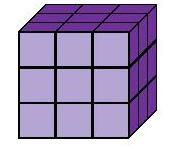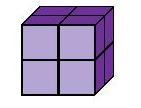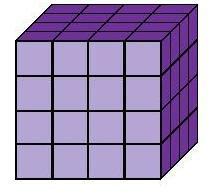### Cubes

When a number is multiplied by itself 3 times it is called a cube:

 13 = 1 × 1 × 1 = 1 33 = 3 × 3 × 3 = 2723 = 2 × 2 × 2 = 8 43 = 4 × 4 × 4 = 64to: